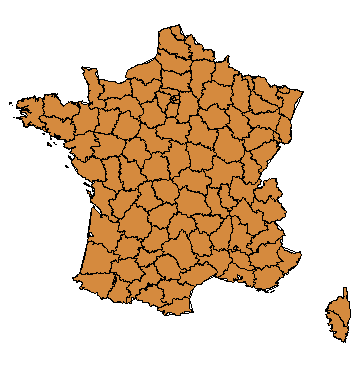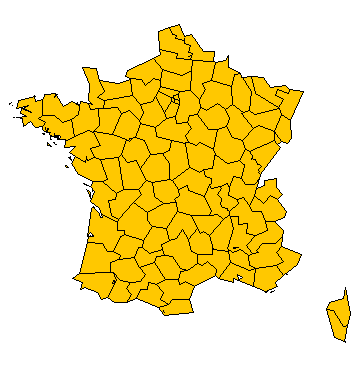# Simplifying a map layer using PostGIS topologyFollowing a recent research about how to simplify a multipolygon layer while keeping topological relationships intact, here’s my take on that, using the PostGIS topological support.

### The data

French administrative subdivisions, called “départements”, will be used. Data can be downloaded here.

It is composed by 96 multipolygons for a total of  47036 vertices.

### Principle of simplification

• We convert a layer’s Geometries to TopoGeometries
• We simplify all edges of the built topology
• We convert the (now-simplified) TopoGeometries back to Geometries

### Steps

The following steps assume you loaded the shapefile into a table named “france_dept”.

```-- Create a topology
SELECT CreateTopology('france_dept_topo', find_srid('public', 'france_dept', 'geom'));

-- Add a layer
SELECT AddTopoGeometryColumn('france_dept_topo', 'public', 'france_dept', 'topogeom', 'MULTIPOLYGON');

-- Populate the layer and the topology
UPDATE france_dept SET topogeom = toTopoGeom(geom, 'france_dept_topo', 1); -- 8.75 seconds

-- Simplify all edges up to 10000 units
SELECT SimplifyEdgeGeom('france_dept_topo', edge_id, 10000) FROM france_dept_topo.edge; -- 3.86 seconds

-- Convert the TopoGeometries to Geometries for visualization
ALTER TABLE france_dept ADD geomsimp GEOMETRY;
UPDATE france_dept SET geomsimp = topogeom::geometry; -- 0.11 seconds```

### The SimplifyEdgeGeom function

You may have noticed that the “SimplifyEdgeGeom” is not a core function. It is a function I wrote for the purpose of catching topological problems introduced by simplification.

The naive call would be:

```SELECT ST_ChangeEdgeGeom('france_dept_topo', edge_id, ST_Simplify(geom, 10000))
FROM france_dept_topo.edge;```

The problem with the above call is that any simplification introducing a topology error would be rejected by ST_ChangeEdgeGeom by throwing an exception and the exception would rollback the whole transaction leaving you with no edge changed. Possible topology errors introduced are: edges collapsing to points, intersecting self or other edges.

The SimplifyEdgeGeom function wraps the ST_ChangeEdgeGeom call into a subtransaction and handles exceptions by reducing the simplification factor until it succeeds. The version I used reduces simplification factor in half at each failure, dropping down to zero around 1e-8. You can roll your own with other heuristics or generalize this one to take parameters about stepping and limits.

Here’s the function:

```CREATE OR REPLACE FUNCTION SimplifyEdgeGeom(atopo varchar, anedge int, maxtolerance float8)
RETURNS float8 AS \$
DECLARE
tol float8;
sql varchar;
BEGIN
tol := maxtolerance;
LOOP
sql := 'SELECT topology.ST_ChangeEdgeGeom(' || quote_literal(atopo) || ', ' || anedge
|| ', ST_Simplify(geom, ' || tol || ')) FROM '
|| quote_ident(atopo) || '.edge WHERE edge_id = ' || anedge;
BEGIN
RAISE DEBUG 'Running %', sql;
EXECUTE sql;
EXCEPTION
WHEN OTHERS THEN
RAISE WARNING 'Simplification of edge % with tolerance % failed: %', anedge, tol, SQLERRM;
tol := round( (tol/2.0) * 1e8 ) / 1e8; -- round to get to zero quicker
IF tol = 0 THEN RAISE EXCEPTION '%', SQLERRM; END IF;
END;
END LOOP;
END
\$ LANGUAGE 'plpgsql' STABLE STRICT;```

### Performance

The times shown near the “expensive” steps give you an indication of the performance you may expect. It’s about 13 seconds in total for the 3 steps outlined in the first paragraph.

A single run of the simplification step brought vertices down to 1369 (from 47036).

Timings are take on this system:

POSTGIS=”2.0.1SVN r9637″ GEOS=”3.4.0dev-CAPI-1.8.0″ PROJ=”Rel. 4.8.0, 6 March 2012″ GDAL=”GDAL 1.9.0, released 2011/12/29″ LIBXML=”2.7.6″ LIBJSON=”UNKNOWN” TOPOLOGY RASTER

PostgreSQL 8.4.10 on x86_64-pc-linux-gnu, compiled by GCC gcc-4.4.real (Ubuntu 4.4.3-4ubuntu5) 4.4.3, 64-bit

CUSTOM OPTIONS:
shared_buffers = 128MB      (default is 24MB)
temp_buffers = 32MB         (default is 8MB)
work_mem = 8MB              (default is 1MB)
maintenance_work_mem = 32MB (default is 16MB)
max_stack_depth = 4MB       (default is 2MB)
checkpoint_segments = 24    (default is 3)

CPU: Intel(R) Core(TM)2 Duo CPU P9500 @ 2.53GHz (2 x 5054.34 bogomips)

RAM: 4GB

### Considerations

The procedure described in this post is also valid for LINESTRING and MULTILINESTRING layers, using the exactly same code.

You could reuse the topology to produce multiple resolution levels w/out incurring again in the construction cost (and with a reduced input complexity at each level).

The simplification step doesn’t use TopoGeometry objects at all so you could choose to perform  topology construction and  attribute assignment in a different way.

Running the SimplifyEdgeGeom function again might give you more simplification because edges which may have intersected to the simplified version of an edge may not be intersecting anymore after their own simplification. The function can be changed to behave differently on exception to improve performance or quality.